# Kronecker symbol

(diff) ← Older revision | Latest revision (diff) | Newer revision → (diff)

The numberdefined by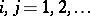. When, the Kronecker symbolhas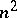components, and the matrix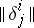is the unit matrix. The Kronecker symbol was first used by L. Kronecker (1866).

The Kronecker symbol may be generalized, considering instead a set of quantities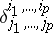withinteger (upper and lower) indices,,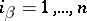, equal to(or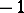) if the sequence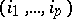is an even (odd) permutation of the distinct indices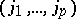and zero otherwise. The numbers(when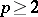often denoted by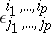) are called the components of the Kronecker symbol. An affine tensor of type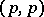whose components relative to some basis are equal to the components of the Kronecker symbol has the same components relative to any other basis.

The Kronecker symbol is convenient in various problems of tensor calculus. For example, the determinant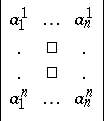is equal to the sum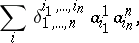where the summation is performed over all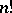permutations of the numbers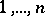. The alternant of the tensor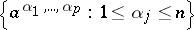is given by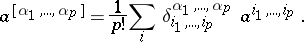How to Cite This Entry:
Kronecker symbol. Encyclopedia of Mathematics. URL: http://encyclopediaofmath.org/index.php?title=Kronecker_symbol&oldid=18809
This article was adapted from an original article by L.P. Kuptsov (originator), which appeared in Encyclopedia of Mathematics - ISBN 1402006098. See original article